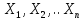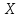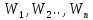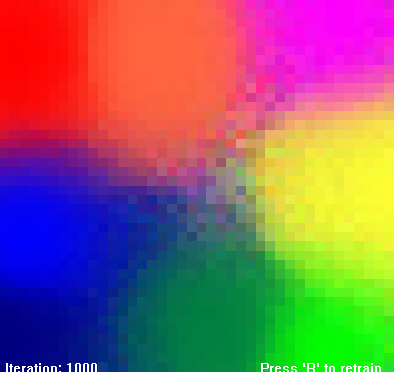Page 2/6 Date 08.07.2018 Size 337.6 Kb.

## 3 Self-Organizing Maps

SOMs consist of nodes or neurons. Each neuron has weight vectors attached to them. The dimensioanlity of the weight vector matches that of the data that the SOM will be trained on. If, where eachis an element of a vector from the training data set, then the corresponding weight vector would be. Like all neural networks, SOMs need to be trained. The goal is to make them act a certan way, given a particular input. This is achieved by adjusting each nodes weight vectors to resemble those in the training data set.

SOMs utilize unsupervized learning. The general algorithm for training a SOM is as follows:

1. Initialize weights of each node

2. Choose a vector from the training data

3. Calculate which node has a weight vector that matches the input vector the best. This is also known as the best matching unit, or the BMU.

4. Calculate the radius of the BMU.

5. Alter the weights of all the nodes in radius of the BMU. This alteration is inversely related to the distance a node has from the BMU. The greater the distance, the less the weights are changed.

6. Adjust the learning rate and go to step 2 for N iterations.

Learning rate is decreased over time. This is to make sure that previous learned inputs aren’t discarded. The algorithm stops when a predefined number of iterations has been completed or the average change of weights per iteration drops below a certain predefined value (this value is from now on referred to as delta). As can be seen, no predefined target vectors are set, so the algortihm requires no other input aside from a set of training data.

The most common demonstration of this algorithm is plotting colors on a 2-dimensional grid. As colors consist of varying values of red, green and blue, the dimensionality of the data is reduced. An example of this is shown in Figure 4.Figure 4 - 8 colors mapped onto a 2D grid by a SOM